Courses

# Butter worth Filter Design Electrical Engineering (EE) Notes | EduRev

## Electrical Engineering (EE) : Butter worth Filter Design Electrical Engineering (EE) Notes | EduRev

The document Butter worth Filter Design Electrical Engineering (EE) Notes | EduRev is a part of the Electrical Engineering (EE) Course Digital Signal Processing.
All you need of Electrical Engineering (EE) at this link: Electrical Engineering (EE)

Butter worth Filter Design

The butterworth LP filter of order N is defined as HB(s) HB(-s) =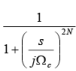Where s = jΩc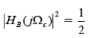OR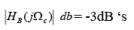It has 2N poles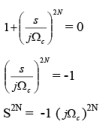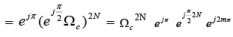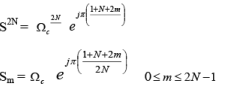Ex: for N=3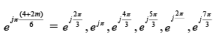= 1200, 1800, 2400, 3000, 3600, 600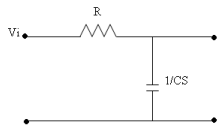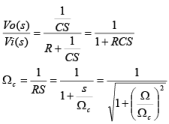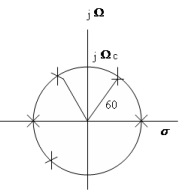Poles that are let half plane are belongs to desired system function.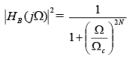For a large Ω, magnitude response decreases as Ω -N, indicating the LP nature of this filter.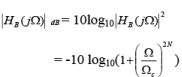As Ω → ∞

= -20 N log10Ω

= -20 N dB/ Decade = -6 N dB/Octane

As N increases, the magnitude response approaches that of ideal LP filter.
The value of N is determined by Pass & stop band specifications.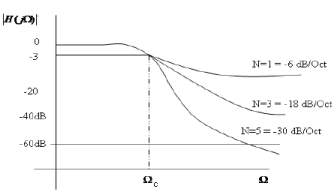Ex: Design Butterworth LPF for the following specifications.

Pass band:

-1< H ( jΩ) 2 dB ≤ 0 for 0≤ Ω ≤ 1404π ( W π = 1404π )

Stop band:

H ( jW)2 dB < -60  for W ≥ 8268π   ( Ωs = 8268π )

If the Ωc is given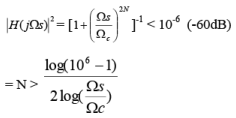Since Ωc is not given, a guess must be made.

The specifications call for a drop of -59dB, In the frequency range from the edge of the pass band (1404π ) to the edge of stop band (8268π ). The frequency difference is equal to

log2(8268/1404)=2.56 octaves.

1 oct ---- - 6N dB

2.56 ------ ?

=> 2.56 X - 6N dB = -59 dB’s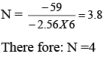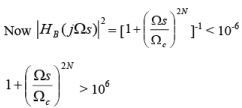Ω2N > 106 Ωc 2 N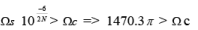Ω c <1470.3π

Let Ω c =1470.3π

At this Ω c it should satisfy pass band specifications.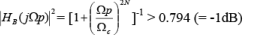= 0.59

This result is below the pass band specifications. Hence N=4 is not sufficient.

Let N=5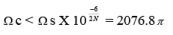In the pass band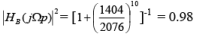Since N=5

Ω c = 2076π

S1 = -2076π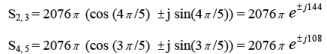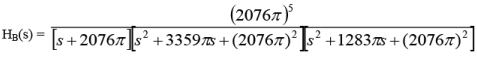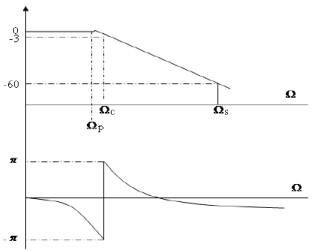1. Magnitude response is smooth, and decreases monotonically as Ω increases from 0 to ∞

2. the magnitude response is maximally flat about Ω =0, in that all its derivatives up to order N are equal to zero at Ω =0

Ex: Ωc=1, N=1

HB( jΩ)2= (1+ Ω 2)-1

The first derivative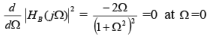The second derivative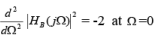3. The phase response curve approaches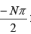for large Ω , where N is the no. of poles of butterworth circle in the left side of s-plane.

1. easiest to design

2. used because of smoothness of magnitude response .

Relatively large transition range between the pass band and stop band.

Other procedure

When Ω c = 1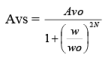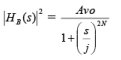If n is even S2N = 1 = e j ( 2k -1)π

The 2N roots will be Sk=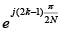k=1,2,….2N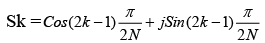Therefore: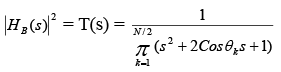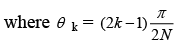If N is odd

S2n =1 = e j 2kπ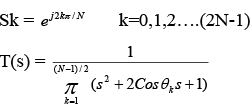where θ k =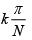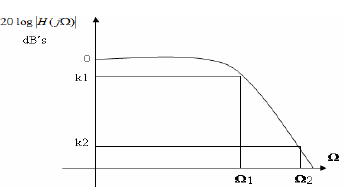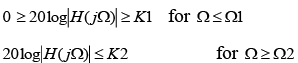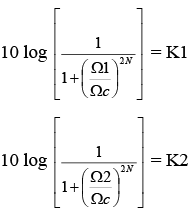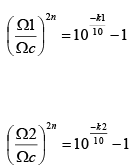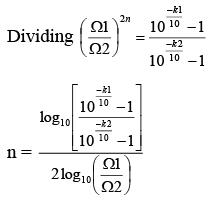choosing this value for n, results in two different selections for Ω. If we wish to satisfy our requirement at Ω1 exactly and do better than our req. at Ω2 , we use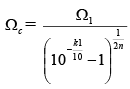or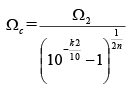for better req at Ω2

Offer running on EduRev: Apply code STAYHOME200 to get INR 200 off on our premium plan EduRev Infinity!

,

,

,

,

,

,

,

,

,

,

,

,

,

,

,

,

,

,

,

,

,

;